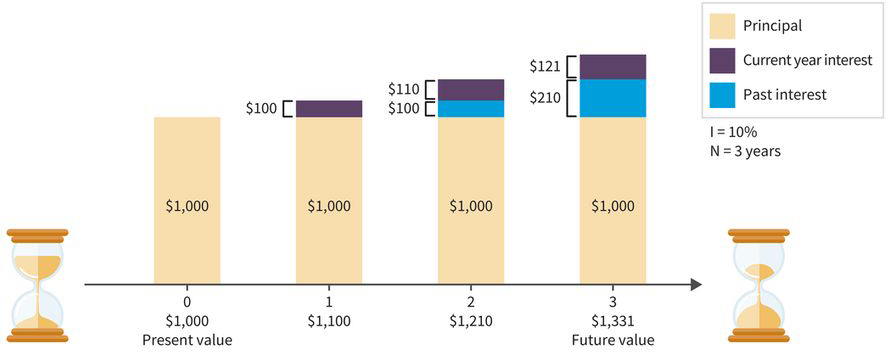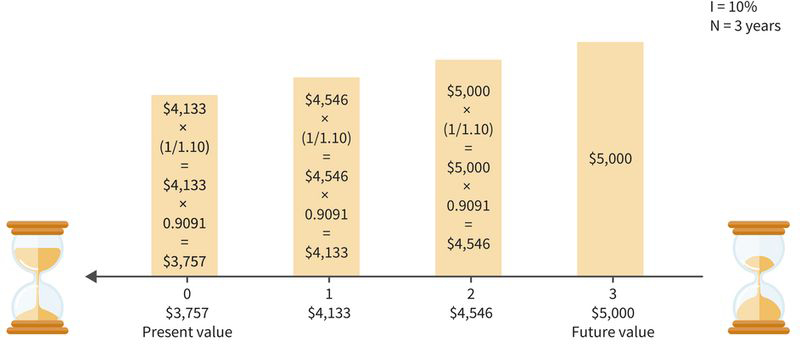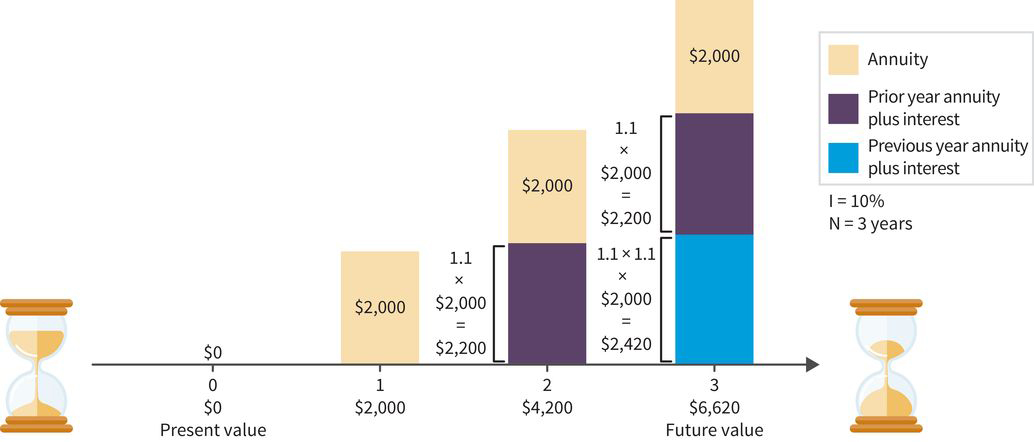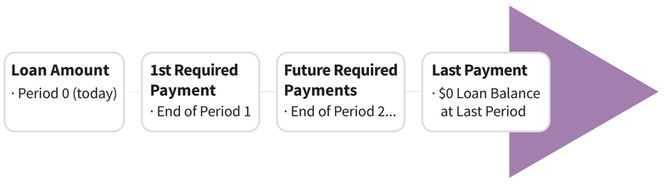+

# Time Value of Money

##### Rating:
(0)
Author: Sophia Tutorial
##### Description:

Determine the appropriate time value of money (TVM) calculation needed to meet a financial goal.

(more)
Tutorial
what's covered
In this tutorial, you will learn how an investment made today will change value in the future based on an applied interest rate. You’ll also learn how much money you need to save (and how often) to reach a future financial goal in the most productive way. Specifically, this lesson will cover:
1. Personal Finance and the Time Value of Money
2. Understanding Time Value of Money (TVM)
3. The Basic TVM Concepts
1. Future Value of a Lump Sum
2. Present Value of a Lump Sum
3. Future Value of an Annuity
4. Amortization or Payment Schedule
4. TVM Shortcut: The Rule of 72

## 1. Personal Finance and the Time Value of Money

During your lifetime, you’ll want your money working for you. A good start is understanding calculations you can use to plan and track your financial progress. The principle of compound growth is a powerful concept that can begin putting your money to work for you. By knowing how to calculate interest, you’ll quickly see the benefit of saving and investing, and understand the cost of borrowing money. When you’re through with this tutorial, you’ll be able to answer questions like:

• Was a recent large purchase worth the money?
• Was it the best way to spend your money at the time?
• Is an investment made today going to be worth it in the long run?
• What factors should you consider when making large purchases and investments?

## 2. Understanding Time Value of Money (TVM)

Once you have defined your financial goals, you need to link each hoped-for outcome with your current financial information. This is where financial tools come into play. Time value of money (TVM) formulas and calculations are valuable personal finance tools because they allow you to specifically consider financial goals in terms of money, time, and interest.

 Productivity: Skill Reflect
Think about a recent financial goal. Did it take you three years to save that money when your financial plan said it should have taken you six months? If so, even if you met your goal, you probably wouldn’t consider it a productive process.

Anytime you are working with a financial goal that involves money, time, and interest, you will want to use TVM calculations. For some students, TVM is a challenging aspect of learning about personal finance topics. This is, after all, the one area that requires the use of math formulas and calculations. But remember – especially if you have a math phobia – that doing these TVM calculations becomes much easier with a little practice.

Most individuals also prefer to use a financial calculator, app, or computer spreadsheet program to solve TVM problems. Financial calculators or TVM apps are easy to use and simplify the math. We will include some basic instructions for using a calculator to solve TVM problems in another topic.

term to know

Time Value of Money (TVM)
Formulas and calculations that allow you to specifically consider financial goals in terms of money, time, and interest.

## 3. The Basic TVM Concepts

Let’s start with a basic outline of four common types of TVM problems. These are the tools that you’ll use to measure your financial goals:

concept to know

All TVM calculations assume compound interest.
• Future value of a lump sum. Estimates how much current savings and investments will be worth at a certain date in the future.
• Present value of a lump sum. Determines the current value of a future amount.
• Future value of an annuity. Estimates how much you will have in the future if you save or invest a set dollar amount on a regular basis.
• Amortization or payment schedule. Calculates the payment you need to make on a car, home, education, or similar type of loan.
3a. Future Value of a Lump Sum
The illustration below shows the timeline associated with calculating a future value of a lump sum. If you start with \$1,000 today, how much will you have in 3 years if you can earn 10% each year? In other words, what is the future value of this amount?

### IN CONTEXT

• PV = \$1,000: You know what you have today (\$1,000). This is called the present value (PV).
• I = 10%: you know the rate of return (I) of what you can earn on your money each year (10%).
• N = 3: you know the number of periods (N) in which you will earn your return (3 years).
• FV = ?: you are trying to find the future value (FV), which is what you will have at the end of the 3-year period.

We’ll show you in another topic how you can use a TVM formula, calculator, or table to determine the future value of a lump sum. But for now, you can get this answer by multiplying \$1,000 by (1.10 × 1.10 × 1.10), which equals \$1,331. Notice that when you are looking for a future value, you multiply your present value by a factor greater than 1.0.Future Value of a Lump Sum Timeline.

terms to know

Future Value of a Lump Sum
An estimate of the value of a single dollar amount at some future date given a known interest rate and number of periods.
Present Value (PV)
An estimate of the current value of a lump-sum amount and/or a series of payments given a known discount rate (or interest rate) and number of periods.
Rate of Return (I)
The amount you can earn on your money each year.
Number of Periods (N)
The amount of compounding periods used in time value of money calculations.
Future Value (FV)
The amount to be earned at the end of a specific period of time.
3b. Present Value of a Lump Sum
The illustration below shows the timeline when calculating a present value of a lump sum, which is the value now of a given amount to be paid or received in the future, assuming compound interest.

hint
You might use a present value calculation when you know how much you will need in the future (FV) but you are unsure if the amount you have today (PV) is going to be enough given your time horizon (N) and rate of return (I).

### IN CONTEXT

• For example, how much do you need today (PV) if you know that you will need \$5,000 (FV) in 3 years (N) assuming you can earn 10% interest (I)?
• With these inputs and working backward, you can determine that you need \$3,757 in hand today (PV) to reach your goal.
• This process of determining the present value is called discounting the future value.Present Value of a Lump Sum Timeline.

We’ll show you in another topic how to use a TVM formula to determine the present value of a lump sum, but for now you can get this answer by multiplying \$1,000 by ([1/1.10] × [1/1.10] × [1/1.10]) or \$1,000 by (0.9091 × 0.9091 × 0.9091). Notice that when finding the present value, you multiply the future amount by a factor that is less than 1.0.

terms to know

Present Value of a Lump Sum
The value now of a given amount to be paid or received in the future, assuming compound interest.
Discounting
Process of determining ­the present value from the amount to be paid or received in the future.
3c. Future Value of an Annuity
The illustration below shows the timeline involved when calculating a future value of an annuity, which is the sum of all the payments (receipts) plus the accumulated compound interest on the payments. Recall that an annuity is a series of equal payments.

hint
Annuity payments can be contributions to an account or distributions from an account.

### IN CONTEXT

• For example, assume you are investing \$2,000 (PMT) at the end of each year for 3 years (N) at a rate of return (I) of 10%. The present value (PV) is \$0, which means that there is no other money in the account at the start.
• With these inputs, at the end of the compounding period, you’ll have a future value (FV) of \$6,620.

We’ll show you in another topic how to use a TVM formula to determine the future value of an annuity, but for now you can get this answer by using the following calculation (each period is represented in parentheses): (2,000) + (1.1 × 2,000) + (1.1 × 1.1 × 2,000).Future Value of an Annuity Timeline.

terms to know

Future Value of an Annuity
The sum of all the payments (receipts) plus the accumulated compound interest on the payments.
Annuity
A series of equal payments.
3d. Amortization or Payment Schedule
The illustration below shows the timeline associated with an amortization schedule.
• The process of amortization involves paying off a current loan or debt, such as a mortgage or car loan, using a series of payments over time.
• At the end of the time period, the loan value will be zero.Loan Amortization Timeline.

When calculating the payment needed to pay off a loan (PMT), you need to know the number of payments (N), the interest being paid (I), and the starting loan amount (PV).

### IN CONTEXT

Say you want to purchase a car with a loan. How would you calculate the amortization schedule using TVM?
• The amortization calculation will tell you how much you need to pay per month to pay off the loan.
• To do this calculation correctly, you must be specific when choosing the number of payments. For example, most home, automobile, and student loans are amortized on a monthly basis. This means that if you have a 5-year loan, you’ll actually be making 60 payments.
• The interest input needs to be adjusted. If your loan APR is 6%, then you are really paying 0.50% (6% ÷ 12) per month in interest.

The math for this calculation is a bit more involved, but we’ll show you a shortcut for doing a payment calculation next.

term to know

Amortization
The process of paying off a current loan or debt, such as a mortgage or a car loan, using a series of payments over time.

## 4. TVM Shortcut: The Rule of 72

Perhaps the simplest “shortcut” to TVM calculations is the Rule of 72.

formula

The Rule of 72
• To find the approximate number of years (or periods) needed to double your money, just divide 72 by the interest rate (or 72/I).
• To estimate the approximate interest rate needed to double your money, divide 72 by the number of years (or periods) in your goal time horizon (or 72/N).

EXAMPLE

Let’s say you want to estimate how many years it will take your money to grow from \$3,000 to \$6,000 if you can earn 3% interest.
• Using the Rule of 72, divide 72 by 3 (not 0.03).
• Did you get 24? The actual answer using TVM calculations is 23.45, but 24 is a close estimate.

EXAMPLE

Now let’s say you want to know what interest rate you need to earn to turn \$3,000 into \$6,000 over 6 years.
• Using the Rule of 72, divide 72 by 6.
• The answer is 12%, which is close to the TVM calculation of 12.25%.

As shown in the table below, the Rule of 72 works nicely as a way to approximate how long it will take to double your money for any financial goal. The higher the interest rate, within reason, the more accurate the Rule of 72 becomes. In situations in which the interest rate is very low – say, below 5% – then you might want to substitute 70 for 72.

Rate Actual Periods Rule of 72 Estimate Rule of 70 Estimate
1.00% 69.66 72.00 70.00
2.00% 35.00 36.00 35.00
3.00% 23.45 24.00 23.33
4.00% 17.67 18.00 17.50
5.00% 14.21 14.40 14.00
6.00% 11.90 12.00 11.67
7.00% 10.24 10.29 10.00
8.00% 9.01 9.00 8.75
9.00% 8.04 8.00 7.78
10.00% 7.27 7.20 7.00
11.00% 6.64 6.55 6.36
12.00% 6.12 6.00 5.83
13.00% 5.67 5.54 5.38
14.00% 5.29 5.14 5.00
15.00% 4.96 4.80 4.67

### IN CONTEXT

Jared is saving for a down payment to purchase a house. Based on his budget, he can save \$4,500 per year while earning a 5% rate of return (APY). How much will Jared have for a down payment at the end of 5 years? Use the mathematical processes demonstrated in this topic, or you can use an online TVM calculator or app, to determine the answer.

The inputs are PV (\$4,500), N (5 years), and I (5%). The answer (output, or FV) is \$24,865.34. You can estimate this amount by using the following calculation (each period is represented in parentheses): (4,500) + (1.05 × 4,500) + (1.05 × 1.05 × 4,500) + (1.05 × 1.05 × 1.05 × 4,500) + (1.05 × 1.05 × 1.05 × 1.05 × 4,500).

summary
Understanding the time value of money (TVM) is important when you’re earning interest on an investment, saving money for a large purchase, or making a series of payments on a loan. Decisions you make in these situations affect your personal finances based on the principle of compound growth. There are several basic TVM concepts:
• Future value of a lump sum. Example: Estimating the future value of your savings account after it accrues interest.
• Present value of a lump sum. Example: Estimating how much money you need to invest today so it reaches a desired amount years from now.
• Future value of an annuity. Example: Estimating how much money you’ll have for a large purchase if you invest the same amount each month.
• Amortization or payment schedule. Example: Estimating your monthly payment on a loan to determine how long it will take to pay it off.

To determine how many periods it will take your investments to double at a fixed interest rate, use the TVM shortcut, the Rule of 72. You can also use this rule to find the interest rate needed to double your money if the number of periods is known. Understanding these concepts can help you improve your productivity skill which, in turn, can help you achieve your goals sooner.

Source: This content has been adapted from Chapter 2.2 of Introduction to Personal Finance: Beginning Your Financial Journey. Copyright © 2019 John Wiley & Sons, Inc. All rights reserved. Used by arrangement with John Wiley & Sons, Inc.

Wiley and the Wiley logo are trademarks or registered trademarks of John Wiley & Sons, Inc. and/or its affiliates in the United States and other countries.

Terms to Know
Amortization

The process of paying off a current loan or debt, such as a mortgage or a car loan, using a series of payments over time.

Annuity

A series of equal payments.

Discounting

Process of determining ­the present value from the amount to be paid or received in the future.

Future Value (FV)

The amount to be earned at the end of a specific period of time.

Future Value of a Lump Sum

An estimate of the value of a single dollar amount at some future date given a known interest rate and number of periods.

Future Value of an Annuity

The sum of all the payments (receipts) plus the accumulated compound interest on the payments.

Number of Periods (N)

The amount of compounding periods used in time value of money calculations.

Present Value (PV)

An estimate of the current value of a lump-sum amount and/or a series of payments given a known discount rate (or interest rate) and number of periods.

Present Value of a Lump Sum

The value now of a given amount to be paid or received in the future, assuming compound interest.

Rate of Return (I)

The amount you can earn on your money each year.

Time Value of Money (TVM)

Formulas and calculations that allow you to specifically consider financial goals in terms of money, time, and interest.

Rating Header